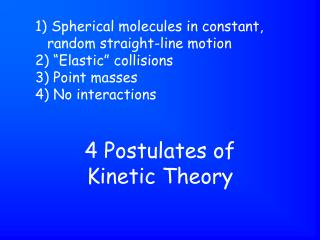Download Presentation4 Postulates of Kinetic Theory

# 4 Postulates of Kinetic Theory - PowerPoint PPT PresentationDownload Presentation## 4 Postulates of Kinetic Theory

- - - - - - - - - - - - - - - - - - - - - - - - - - - E N D - - - - - - - - - - - - - - - - - - - - - - - - - - -
##### Presentation Transcript

1. Spherical molecules in constant, random straight-line motion • “Elastic” collisions • Point masses • No interactions 4 Postulates of Kinetic Theory

2. Gas that obeys all 4 assumptions of the kinetic theory all of the time. It doesn’t exist. It’s a model. Ideal Gas

3. Spherical molecules in random, straight-line motion • “Elastic” collisions Which assumptions of the kinetic theory hold up?

4. Point masses • No interactions Which assumptions of the kinetic theory break down?

5. When the gas molecules are close to each other. When do the assumptions of the kinetic theory break down?

6. At high pressure & low temperature. When are the gas molecules close to each other?

7. At low pressure & high temperature. When are the gas molecules far apart from each other?

8. Good! How do the gas molecules act when they are far apart from each other?

9. Have mass • Take the shape & volume of their container • Compressible • Diffuse (spread spontaneously) • Exert Pressure What are the properties of gases?

10. Force/Area Pressure

11. Collisions of the gas molecules with the walls of the container. Pressure results from?

12. # of impacts per unit time and force of each impact Pressure depends on? (microscopically)

13. # of gas molecules per unit volume And temperature Pressure depends on? (macroscopically)

14. 1 atm = 760 mm Hg = 760 torr = 101.3 kPa Units of Pressure?

15. P = 1 atm T = 0C = 273 K STP

16. The volume of 1 mole of any gas at STP = 22.4 Liters Molar Volume at STP

17. A measure of the avg. kinetic energy of the particles of a substance. Temperature

18. Temperature • Pressure • Volume • # of moles 4 variables needed to completely describe a gas-phase system?

19. For a fixed mass and temperature, the pressure-volume product is a constant. Boyle’s Law

20. PV = k where k = a constant Boyle’s Law

21. P1V1 = P2V2 Boyle’s Law

22. Hyperbola – it’s an inverse relationship! Graph of Boyle’s Law

23. Graph of Boyle’s Law, Pressure vs. Volume

24. Volume goes to ½ the original volume Double the pressure

25. Volume goes to 1/3 the original volume Triple the pressure

26. Volume goes to 2 X the original volume Halve the pressure

27. Volume goes to 1/4 of the original volume Quadruple the pressure

28. What does the graph of a direct relationship look like?

29. Kelvin: 0 K means 0 speed. Which temperature scale has a direct relationship to molecular velocity?

30. Kelvin: 0 K means 0 speed. Absolute Temperature Scale

31. It’s a direct relationship. Graph of Volume vs. Kelvin Temperature

32. V1/T1 = V2/T2 Charles’ Law Math expression of relationship between volume & Kelvin temperature

33. Volume of a gas varies directly with the Kelvin temperature. Verbal expression of Charles’ Law

34. The volume doubles! What happens to the volume when the Kelvin temperature is doubled?

35. The volume triples! What happens to the volume when the Kelvin temperature is tripled?

36. The volume is halved! What happens to the volume when the Kelvin temperature is halved?

37. It’s halved! What happens to the Kelvin temperature when the volume is halved?

38. It’s a direct relationship. Graph of Pressure vs. Kelvin Temperature

39. P1/T1 = P2/T2 Gay-Lussac’s Law Math expression for relationship between pressure & Kelvin temperature.

40. The pressure of a gas varies directly with the Kelvin temperature. Verbal expression of relationship between pressure & Kelvin temperature.

41. The pressure is doubled. What happens to the pressure when the Kelvin temperature is doubled?

42. The pressure is halved. What happens to the pressure when the Kelvin temperature is halved?

43. The pressure is tripled. What happens to the pressure when the Kelvin temperature is tripled?

44. The Kelvin temperature is doubled. What happens to the Kelvin temperature when the pressure is doubled?

45. P1V1 = P2V2 T1 T2 Same pressure & volume units on both sides. Temperature must be in Kelvins. Combined Gas Law

46. Equal volumes of gases at the same temperature & pressure have equal numbers of molecules. What is Avogadro’s Principle?

47. He N2 These 2 boxes have the same: Mass c) # of molecules Density d) # of atoms

48. Ptot = P1 + P2 + P3 + P4 + … What is Dalton’s Law of Partial Pressures?